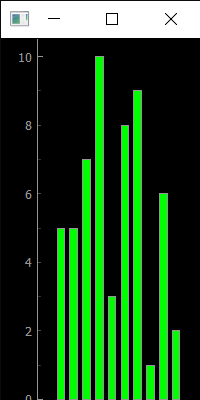# PyQtGraph – Resize Plot Window

• Last Updated : 24 Sep, 2020

In this article we will see how we can resize the plot window in the PyQtGraph module. PyQtGraph is a graphics and user interface library for Python that provides functionality commonly required in designing and science applications. Its primary goals are to provide fast, interactive graphics for displaying data (plots, video, etc.) and second is to provide tools to aid in rapid application development (for example, property trees such as used in Qt Designer).Plot windows consist of two main parts: the Plot Panel containing the actual plotted graphics and the Control Panel. We can set the window geometry with the help of `setGeometry` method, but it is used to specify the position as well although we can only set or change the size of the window by resizing it programmatically.

We can create a plot window with the help of command given below

```# creating a pyqtgraph plot window
window = pg.plot()
```

In order to do this we use `resize` method with the plot window object

Syntax : window.resize(width, height)

Argument : It takes two integer as argument

Return : It returns None

Below is the implementation

 `# importing pyqtgraph as pg``import` `pyqtgraph as pg`` ` `# importing QtCore and QtGui from the pyqtgraph module``from` `pyqtgraph.Qt ``import` `QtCore, QtGui`` ` `# importing numpy as np``import` `numpy as np`` ` `import` `time`` ` `# creating a pyqtgraph plot window``window ``=` `pg.plot()`` ` `# setting window geometry``# left = 100, top = 100``# width = 600, height = 500``window.setGeometry(``100``, ``100``, ``600``, ``500``)`` ` `# title``title ``=` `"GeeksforGeeks PyQtGraph"`` ` `# setting window title``window.setWindowTitle(title)`` ` `# create list for y-axis``y1 ``=` `[``5``, ``5``, ``7``, ``10``, ``3``, ``8``, ``9``, ``1``, ``6``, ``2``]`` ` `# create horizontal list i.e x-axis``x ``=` `[``1``, ``2``, ``3``, ``4``, ``5``, ``6``, ``7``, ``8``, ``9``, ``10``]`` ` `# create pyqt5graph bar graph item ``# with width = 0.6``# with bar colors = green``bargraph1 ``=` `pg.BarGraphItem(x ``=` `x, height ``=` `y1, width ``=` `0.6``, brush ``=``'g'``)`` ` `# add item to plot window``# adding bargraph item to the window``window.addItem(bargraph1)`` ` `# resizing the plot window``window.resize(``200``, ``400``)`` ` ` ` ` ` ` ` `# main method``if` `__name__ ``=``=` `'__main__'``:``     ` `    ``# importing system``    ``import` `sys``     ` `    ``# Start Qt event loop unless running in interactive mode or using ``    ``if` `(sys.flags.interactive !``=` `1``) ``or` `not` `hasattr``(QtCore, ``'PYQT_VERSION'``):``        ``QtGui.QApplication.instance().exec_()``        `

Output :My Personal Notes arrow_drop_up